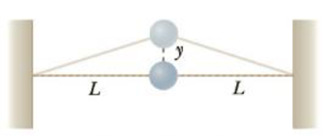Chapter 13, Problem 72AP

Chapter
Section
Textbook Problem

An object of mass m is connected to two rubber bands of length L, each under tension F, as in Figure P13.72. The object is displaced vertically by a small distance y. Assuming the tension does not change, show that (a) the restoring force is −(2F/L)y and (b) the system exhibits simple harmonic motion with an angular frequency ω = 2 F / m L .Figure P13.72

a)

To determine
The restoring force exerted on the rubber band.

Explanation

Given info: The length of the two rubber bands are L . The rubber bands are under tension F . The mass of the object is m . The object is given a vertical displacement of y .

The system is shown in the following figure.

Consider the downward direction as negative. From the above figure, the restoring force on the rubber band is,

Fnet=2Fsinθ (1)

For small displacements

sinθ=tanθ (2)

Moreover the value of <

b)

To determine
The angular frequency of the simple harmonic motion.

Still sussing out bartleby?

Check out a sample textbook solution.

See a sample solution

The Solution to Your Study Problems

Bartleby provides explanations to thousands of textbook problems written by our experts, many with advanced degrees!

Get Started

People most often choose foods for the nutrients they provide. T F

Nutrition: Concepts and Controversies - Standalone book (MindTap Course List)

Why can’t a white dwarf have a mass greater than 1.4 solar masses?

Horizons: Exploring the Universe (MindTap Course List)

What gives amino acids their individual properties?

Biology: The Dynamic Science (MindTap Course List)

Match the term listed in Column A with its definition from Column B

Nutrition Through the Life Cycle (MindTap Course List)

What is an antimicrobial?

General, Organic, and Biological Chemistry

With ______, one species evolves to look like another.

Biology: The Unity and Diversity of Life (MindTap Course List)